## 0x01 问题重现

``````let re = /[a-z]+/g;
console.log(re.test("abcde"));
console.log(re.test("abcde"));
console.log(re.test("abcde"));
console.log(re.test("abcde"));
console.log(re.test("abcde"));
console.log(re.test("abcde"));
console.log(re.test("abcde"));
console.log(re.test("abcde"));
console.log(re.test("abcde"));
``````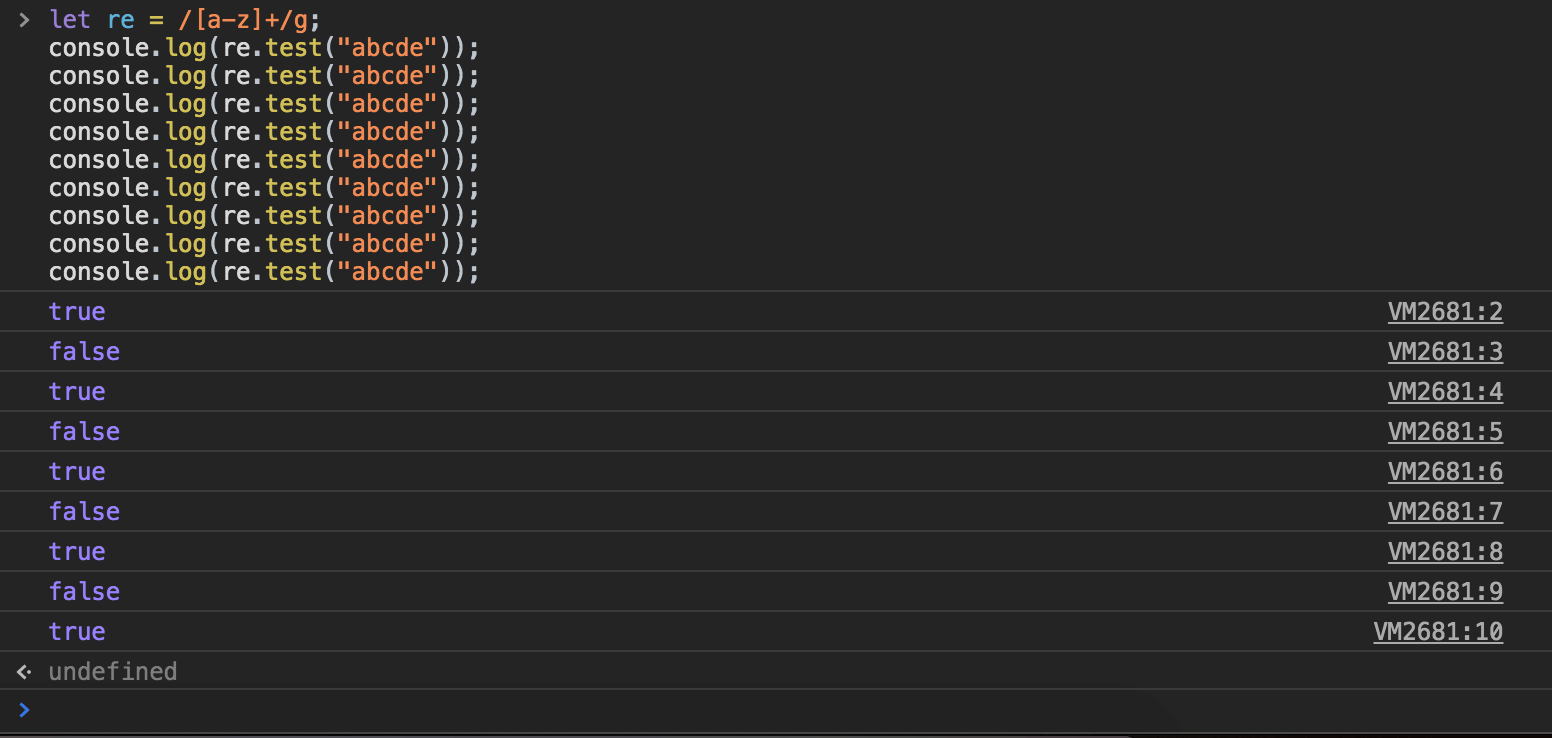## 0x03 阅读源码

``````JS_FN("test", regexp_test, 1,0)
↓
bool js::regexp_test(JSContext *cx, unsigned argc, Value *vp);
↓
static bool regexp_test_impl(JSContext *cx, CallArgs args);
↓
static RegExpRunStatus ExecuteRegExp(JSContext *cx, CallArgs args, MatchPairs &matches)；
↓
RegExpRunStatus js::ExecuteRegExp(JSContext *cx, HandleObject regexp, HandleString string,
MatchPairs &matches, RegExpStaticsUpdate staticsUpdate);
``````

``````RegExpRunStatus
js::ExecuteRegExp(JSContext *cx, HandleObject regexp, HandleString string,
MatchPairs &matches, RegExpStaticsUpdate staticsUpdate)
{
/* Step 1 (b) was performed by CallNonGenericMethod. */
Rooted<RegExpObject*> reobj(cx, &regexp->as<RegExpObject>());

RegExpGuard re(cx);
if (!reobj->getShared(cx, &re))
return RegExpRunStatus_Error;

RegExpStatics *res;
if (staticsUpdate == UpdateRegExpStatics) {
res = cx->global()->getRegExpStatics(cx);
if (!res)
return RegExpRunStatus_Error;
} else {
res = nullptr;
}

/* Step 3. */
RootedLinearString input(cx, string->ensureLinear(cx));
if (!input)
return RegExpRunStatus_Error;

/* Step 4. */
RootedValue lastIndex(cx, reobj->getLastIndex());
size_t length = input->length();

/* Step 5. */
int i;
if (lastIndex.isInt32()) {
/* Aggressively avoid doubles. */
i = lastIndex.toInt32();
} else {
double d;
if (!ToInteger(cx, lastIndex, &d))
return RegExpRunStatus_Error;

/* Inlined steps 6, 7, 9a with doubles to detect failure case. */
if (reobj->needUpdateLastIndex() && (d < 0 || d > length)) {
reobj->zeroLastIndex();
return RegExpRunStatus_Success_NotFound;
}

i = int(d);
}

/* Steps 6-7 (with sticky extension). */
if (!re->global() && !re->sticky())
i = 0;

/* Step 9a. */
if (i < 0 || size_t(i) > length) {
reobj->zeroLastIndex();
return RegExpRunStatus_Success_NotFound;
}

/* Steps 8-21. */
size_t lastIndexInt(i);
RegExpRunStatus status = ExecuteRegExpImpl(cx, res, *re, input, &lastIndexInt, matches);
if (status == RegExpRunStatus_Error)
return RegExpRunStatus_Error;

/* Steps 9a and 11 (with sticky extension). */
if (status == RegExpRunStatus_Success_NotFound)
reobj->zeroLastIndex();
else if (reobj->needUpdateLastIndex())
reobj->setLastIndex(lastIndexInt);

return status;
}
``````

``````    #include <iostream>

/* ... */

/* Steps 9a and 11 (with sticky extension). */
if (status == RegExpRunStatus_Success_NotFound)
reobj->zeroLastIndex();
else if (reobj->needUpdateLastIndex())
reobj->setLastIndex(lastIndexInt);

std::cout << reobj->getLastIndex().toInt32() << "\n";

return status;
``````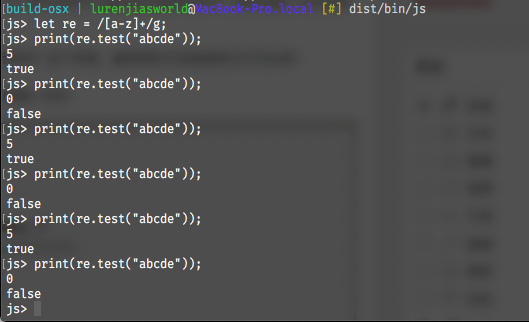## 0x04 分析源码

``````let re = /[a-z]+/g;
let str = "abcde"

// match 1
lastIndex = 0
abcde
_____^_____
lastIndex = 5
return true

// match 2
lastIndex = 5
abcde
______^____
lastIndex = ? // 无法匹配到下一个满足正则表达式的串
=> lastIndex = 0;
return false;

// match 3
lastIndex = 0;
abcde
_____^_____
lastIndex = 5
return true

......
``````

## 0x05 深入理解

``````bool global() const     { return getFixedSlot(GLOBAL_FLAG_SLOT).toBoolean(); }
bool sticky() const     { return getFixedSlot(STICKY_FLAG_SLOT).toBoolean(); }
``````

``````static const unsigned LAST_INDEX_SLOT          = 0;
static const unsigned SOURCE_SLOT              = 1;
static const unsigned GLOBAL_FLAG_SLOT         = 2;
static const unsigned IGNORE_CASE_FLAG_SLOT    = 3;
static const unsigned MULTILINE_FLAG_SLOT      = 4;
static const unsigned STICKY_FLAG_SLOT         = 5;
``````

``````RegExp.prototype.lastIndex
RegExp.prototype.source
RegExp.prototype.global
RegExp.prototype.ignoreCase
RegExp.prototype.multiline
RegExp.prototype.sticky
``````

``````// js/src/vm/RegExpObject.h
// ...
bool ignoreCase() const { return getFixedSlot(IGNORE_CASE_FLAG_SLOT).toBoolean(); }
bool global() const     { return getFixedSlot(GLOBAL_FLAG_SLOT).toBoolean(); }
bool multiline() const  { return getFixedSlot(MULTILINE_FLAG_SLOT).toBoolean(); }
bool sticky() const     { return getFixedSlot(STICKY_FLAG_SLOT).toBoolean(); }
// ...
``````

``````bool ignoreCase() const { return getFixedSlot(IGNORE_CASE_FLAG_SLOT).toBoolean(); }
bool global() const     {
std::cout << std::left << std::setw(24) << "LAST_INDEX_SLOT"        << std::left << std::setw(10) << getFixedSlot(0).toBoolean() << "\n";
std::cout << std::left << std::setw(24) << "SOURCE_SLOT"            << std::left << std::setw(10) << getFixedSlot(1).toBoolean() << "\n";
std::cout << std::left << std::setw(24) << "GLOBAL_FLAG_SLOT"       << std::left << std::setw(10) << getFixedSlot(2).toBoolean() << "\n";
std::cout << std::left << std::setw(24) << "IGNORE_CASE_FLAG_SLOT"  << std::left << std::setw(10) << getFixedSlot(3).toBoolean() << "\n";
std::cout << std::left << std::setw(24) << "MULTILINE_FLAG_SLOT"    << std::left << std::setw(10) << getFixedSlot(4).toBoolean() << "\n";
std::cout << std::left << std::setw(24) << "STICKY_FLAG_SLOT"       << std::left << std::setw(10) << getFixedSlot(5).toBoolean() << "\n";
return getFixedSlot(GLOBAL_FLAG_SLOT).toBoolean();
}
bool multiline() const  { return getFixedSlot(MULTILINE_FLAG_SLOT).toBoolean(); }
bool sticky() const     { return getFixedSlot(STICKY_FLAG_SLOT).toBoolean(); }
``````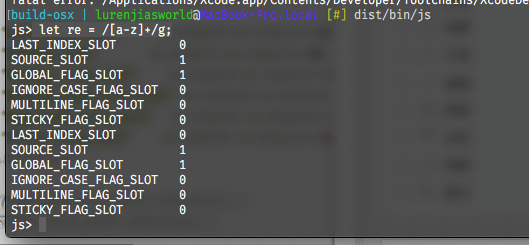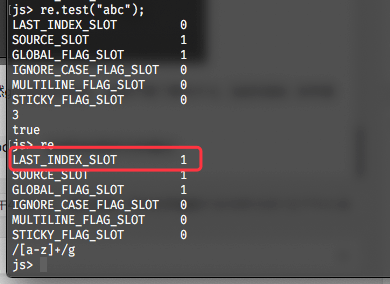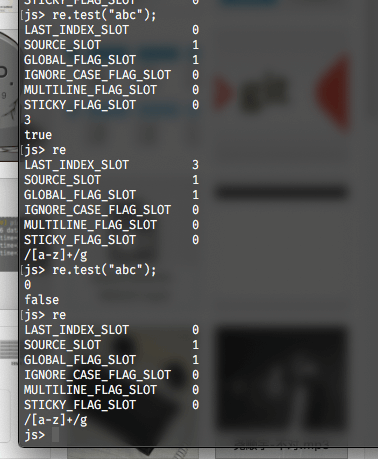global 属性表明正则表达式是否使用了 “g” 标志。global 是一个正则表达式实例的只读属性。

## 0x06 扩展了解

### 1. RegExp.prototype.source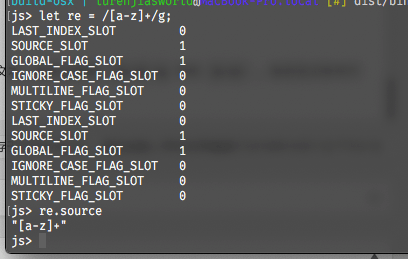### 2. RegExp.prototype.ignoreCase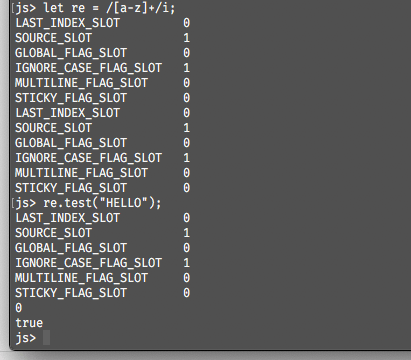### 3. RegExp.prototype.multiline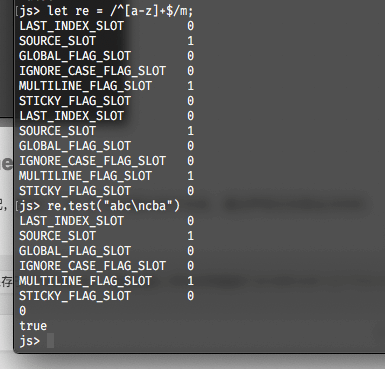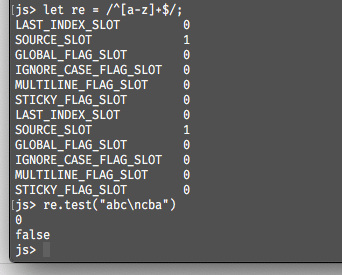### 4. RegExp.prototype.sticky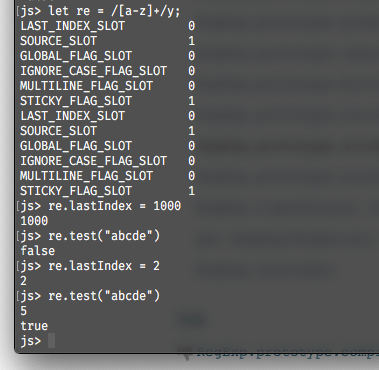## 0x07 参考阅读：V8中关于本文的代码

V8中相关的文件存放于`src/runtime/runtime-regexp.cc`，有`RegExpBuiltinsAssembler::RegExpPrototypeSearchBodySlow``RegExpBuiltinsAssembler::RegExpPrototypeSearchBodyFast`两个实现，用于不同的效率需求。

`RegExpBuiltinsAssembler::RegExpPrototypeSearchBodySlow`举例，我们可以从源码中看到其清晰的匹配流程：

``````void RegExpBuiltinsAssembler::RegExpPrototypeSearchBodySlow(
Node* const context, Node* const regexp, Node* const string) {
CSA_ASSERT(this, IsString(string));

Isolate* const isolate = this->isolate();

Node* const smi_zero = SmiZero();

// Grab the initial value of last index.
Node* const previous_last_index =

// Ensure last index is 0.
{
Label next(this), slow(this, Label::kDeferred);
BranchIfSameValue(previous_last_index, smi_zero, &next, &slow);

BIND(&slow);
SlowStoreLastIndex(context, regexp, smi_zero);
Goto(&next);
BIND(&next);
}

// Call exec.
Node* const exec_result = RegExpExec(context, regexp, string);

// Reset last index if necessary.
{
Label next(this), slow(this, Label::kDeferred);
Node* const current_last_index =

BranchIfSameValue(current_last_index, previous_last_index, &next, &slow);

BIND(&slow);
SlowStoreLastIndex(context, regexp, previous_last_index);
Goto(&next);
BIND(&next);
}

// Return -1 if no match was found.
{
Label next(this);
GotoIfNot(IsNull(exec_result), &next);
Return(SmiConstant(-1));
BIND(&next);
}

// Return the index of the match.
{
Label fast_result(this), slow_result(this, Label::kDeferred);
BranchIfFastRegExpResult(context, exec_result, &fast_result, &slow_result);

BIND(&fast_result);
{
Node* const index =
Return(index);
}

BIND(&slow_result);
{
Return(GetProperty(context, exec_result,
isolate->factory()->index_string()));
}
}
}
``````

## 0x08 参考阅读：SpiderMonkey中曾经存在的一个趣味BUG

BUG描述如下：RegExp类在处理 `^` 断言和 `sticky` 标志时，会允许使用了 `sticky` 标志的表达式从 ^断言开始匹配。此外，这个 bug 是在 Firefox 3.6 之后的某个版本引入的（2010年发布的Firefox版本），并最终于2015年修复。

``````var regex = /^[a-z]+/y;
regex.lastIndex = 2;
regex.test("..foo");
``````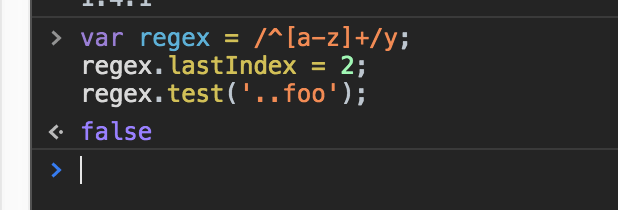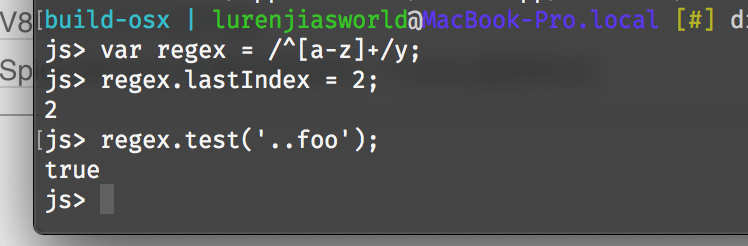NOTE: Even when the y flag is used with a pattern, ^ always matches only at the beginning of Input, or (if Multiline is true) at the beginning of a line.